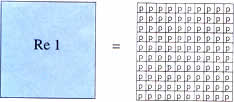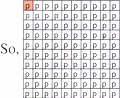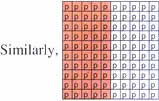Tuesday , July 5 2022# NCERT 5th Class (CBSE) Mathematics: Percentage

### Connecting Percentage and Money

1 rupee = 100 p1 p = 1/100 of a rupee = 1/100 × 100 = 1% of a rupee50 p = 50/100 of a rupee = 50/100 × 100 = 50% of a rupee

### Connecting Percentage and Metric Measures

A. 1 m = 100 cm

So, 1 cm = 1/100 m

= 1/100 × 100 = 1%

1 cm = 1% of a metre

B. 1 kg = 1000 g

1 g = 1/1000 of a kg

As a percentage = 1/1000 × 100 = 0.1%

1 g = 0.1% of a kg

C. l = 1000 ml

So, 1 ml = 1/1000 of a litre

As a percentage = 1/1000 × 100 = 0.1%

1 ml = 0.1% of a litre

## चुनौती हिमालय की 5th NCERT CBSE Hindi Rimjhim Ch 18

चुनौती हिमालय की 5th Class NCERT CBSE Hindi Book Rimjhim Chapter 18 प्रश्न: लद्दाख जम्मू-कश्मीर राज्य में …